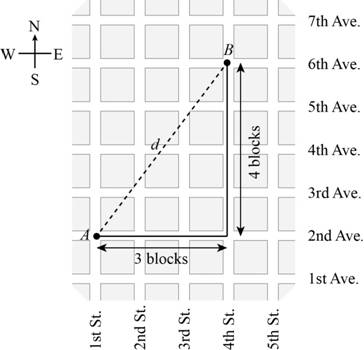# The straight line distance between blocks A and B.### Precalculus: Mathematics for Calcu...

6th Edition
Stewart + 5 others
Publisher: Cengage Learning
ISBN: 9780840068071### Precalculus: Mathematics for Calcu...

6th Edition
Stewart + 5 others
Publisher: Cengage Learning
ISBN: 9780840068071

#### Solutions

Chapter 1.8, Problem 113E

(a)

To determine

## To find: The straight line distance between blocks A and B.

Expert Solution

The straight line distance between blocks A and B is 5 blocks.

### Explanation of Solution

Given:

A city has streets that run north and south and avenues that run east and west, all equally spaced. streets and avenues are numbered sequentially as shown in below figure,Figure (1)

Calculation:

The distance between the points A(x1,y1) and B(x2,y2) in plane is,

(x2x1)2+(y2y1)2

From the Figure (1) it can seen that the distance between A(1,2) and B(4,6) is,

(41)2+(62)2=32+42=9+16=25=5

So the distance between A(1,2) and B(4,6) is 5 blocks.

(b)

To determine

### To find: The walking distance and the straight line distance between the corner of 4th street and 2nd avenue and the corner of 11th street and 26th avenue.

Expert Solution

The walking distance is 7 streets and 24 avenue and the straight line distance is 25 blocks.

### Explanation of Solution

Given:

A city has streets that run north and south and avenues that run east and west, all equally spaced. streets and avenues are numbered sequentially as shown in below figure,Figure (1)

Calculation:

The walking distance is sum of,

11street4street=7street

And

26avenue2avenue=24avenue

Thus the walking distance is 7 streets and 24 avenue.

The straight line distance is the distance between point (4,2) and point (11,26) ,

(114)2+(262)2=72+242=49+576=625=25

So the straight line distance is 25 blocks.

Thus, the walking distance is 7 streets and 24 avenue and the straight line distance is 25 blocks.

(c)

To determine

Expert Solution

### Explanation of Solution

The condition of the walking distance between P and Q is equal to the straight line distance between P and Q, is only possible when the path followed is either only street or only avenue.

### Have a homework question?

Subscribe to bartleby learn! Ask subject matter experts 30 homework questions each month. Plus, you’ll have access to millions of step-by-step textbook answers!